Vectors and conics

This free course is available to start right now. Review the full course description and key learning outcomes and create an account and enrol if you want a free statement of participation.

Free course

4.6 Hyperbola (e > 1)

A hyperbola is the set of points P in the plane whose distances from a fixed point F are e times their distances from a fixed line d, where e > 1. We obtain a hyperbola in standard form if

1. the focus F lies on the x-axis, and has coordinates (ae, 0), where a > 0;

2. the directrix d is the line with equation x = a/e.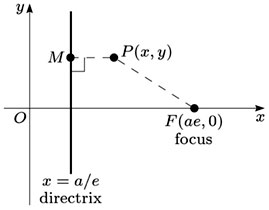Let P (x, y) be an arbitrary point on the hyperbola, and let M be the foot of the perpendicular from P to the directrix. Since FP = e × PM, by the definition of the hyperbola, it follows that FP2 = e2 × PM2; that is,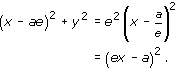Multiplying out the brackets, we obtain

x2 − 2aex + a2e2 + y2 = e2x2 − 2aex + a2,

which simplifies to the equation

x2(e2 − 1) − y2 = a2(e2 − 1),

that is,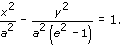Substituting b for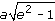, so that b2 = a2(e2 − 1), we obtain the standard form of the equation of the hyperbola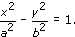This equation is symmetric in x and in y, so that the hyperbola also has a second focus F′ at (−ae, 0), and a second directrix d′ with equation x = −a/e.

The hyperbola intersects the x-axis at the points (±a, 0). We call the line segment joining the points (±a, 0) the major axis or transverse axis of the hyperbola, and the line segment joining the points (0, ±b) the minor axis or conjugate axis of the hyperbola (this is not a chord of the hyperbola). The origin is the centre of this hyperbola.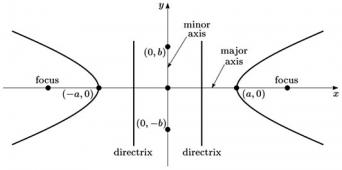Each point with coordinates (a sec t, b tan t) lies on the hyperbola, since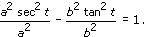Note: In general, sec2t = 1 + tan2t.

Then, just as for the parabola, we can check that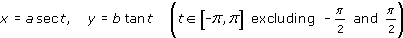gives a parametric representation of the hyperbola.

Note: An alternative parametrisation, using hyperbolic functions, is x = acosht, y = bsinht (t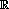).

Two other features of the shape of the hyperbola stand out.

First, the hyperbola consists of two separate curves or branches.

Secondly, the lines with equations y = ±bx/a divide the plane into two pairs of opposite sectors; the branches of the hyperbola lie in one pair. As x → ±∞, the branches of the hyperbola get closer and closer to these two lines. We call the lines y = ±bx/a the asymptotes of the hyperbola.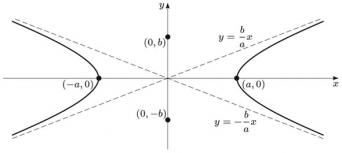We summarise these facts as follows:

Hyperbola in standard form

A hyperbola in standard form has equation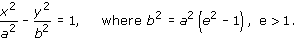It can also be described by the parametric equations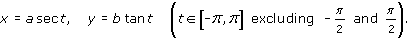It has foci (±ae, 0) and directrices x = ±a/e; its major axis is the line segment joining the points (±a, 0), and its minor axis is the line segment joining the points (0, ±b).

Example 57

Let P be a point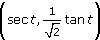, t, on the hyperbola with equation x2 − 2y2 = 1.

• (a) Determine the foci F and F′ of the hyperbola.

• (b) Determine the gradients of FP and FP, when these lines are not parallel to the y-axis.

• (c) Find the point P on the hyperbola, in the first quadrant, for which FP is perpendicular to FP.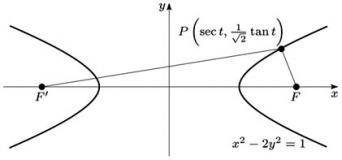• (a) This hyperbola is of the form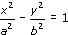with a = 1 and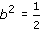, so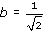. If e denotes the eccentricity of the hyperbola, so that b2 = a2(e2 − 1), we have

•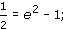• it follows that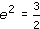, so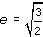• In the general case, the foci are (±ae, 0); it follows that here the foci are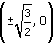.

• (b) Let F and F′ be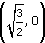and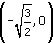, respectively. (It does not matter which way round these are chosen.)

• Then the gradient of FP is

•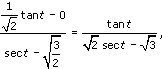• where we know that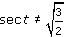, since FP is not parallel to the y-axis.

• Similarly, the gradient of FP is

•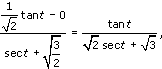• where we know that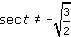, since FP is not parallel to the y-axis.

• (c) When FP is perpendicular to FP, we have

•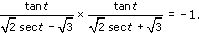• We may rewrite this in the form

•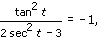• so 2sec2t − 3 + tan2t = 0.

• Since sec2t = 1 + tan2t, it follows that 3 tan2t = 1.

• Since we are looking for a point P in the first quadrant, we choose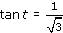.

• When, we have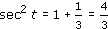. Since we are looking for a point P in the first quadrant, we choose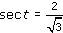.

• It follows that the required point P has coordinates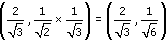.

M208_1